Courses

# Test: Series Circuits And Parallel Networks

## 30 Questions MCQ Test Networking Theory | Test: Series Circuits And Parallel Networks

Description
This mock test of Test: Series Circuits And Parallel Networks for Electrical Engineering (EE) helps you for every Electrical Engineering (EE) entrance exam. This contains 30 Multiple Choice Questions for Electrical Engineering (EE) Test: Series Circuits And Parallel Networks (mcq) to study with solutions a complete question bank. The solved questions answers in this Test: Series Circuits And Parallel Networks quiz give you a good mix of easy questions and tough questions. Electrical Engineering (EE) students definitely take this Test: Series Circuits And Parallel Networks exercise for a better result in the exam. You can find other Test: Series Circuits And Parallel Networks extra questions, long questions & short questions for Electrical Engineering (EE) on EduRev as well by searching above.
QUESTION: 1

### Find the current in the circuit.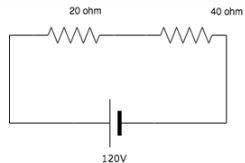Solution:

I = V/R.

Total resistance = 20+40 = 60ohm.

V = 120V.

I = 120/60 = 2A.

QUESTION: 2

### In a series circuit, which of the parameters remain constant across all circuit elements such as resistor, capacitor and inductor?

Solution:

In a series circuit, the current across all elements remain the same and the total volt-age of the circuit is the sum of the voltages across all the elements.

QUESTION: 3

### Voltage across the 60Ω resistor is______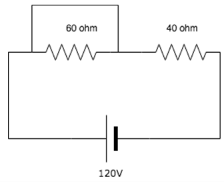Solution:

The 60ohm resistance is shorted since current always choses the low resistance path. So, whole of the current will flow through the alternative route and there will be 0 current through 60Ω resistance.

As,

Voltage across short circuit is equal to zero, hence voltage across the resistor is 0.

QUESTION: 4

Find the voltage across the 6 ohm resistor.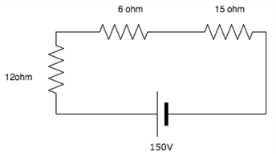Solution:

Total current = 150/(6+12+15)

= 4.55A.

V across 6ohm = Total current x resistance

= 4.55×6

= 27.24V.

QUESTION: 5

If there are two bulbs connected in series and one blows out, what happens to the other bulb?

Solution:

Since the two bulbs are connected in series, if the first bulb burns out there is a break in the circuit and hence the second bulb does not glow.

QUESTION: 6

What is the value of x if the current in the circuit is 5A?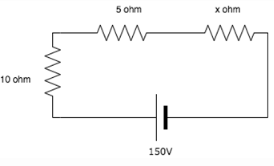Solution:

Total voltage=sum of voltages across each resistor. =>150=10*5+5*5+5*x. Solving the equation, we get x=15 ohm.

QUESTION: 7

Voltage across a series resistor circuit is proportional to?

Solution:

V=IR hence voltage across a series resistor circuit is proportional to the value of the resistance because current remains same in series circuit.

QUESTION: 8

Many resistors connected in series will?

Solution:

In a series circuit, the current remains the same across all resistors hence the voltage divides proportionally among all resistors.

QUESTION: 9

What is the voltage measured across a series short?

Solution:

A short is just a wire. The potential difference between two points of a wire is zero hence the voltage measured is equal to zero.

QUESTION: 10

What happens to the current in the series circuit if the resistance is doubled?

Solution:

Current is inversely proportional to resistance so resistance increased 2 times means current is decreased 2 times

QUESTION: 11

If two bulbs are connected in parallel and one bulb blows out, what happens to the other bulb?

Solution:

In a parallel circuit, if one bulb blows out, it acts as an open circuit. Current does not flow in that branch but it continues to flow in the other branch hence the bulb continues to glow.

QUESTION: 12

Calculate the current across the 20 ohm resistor.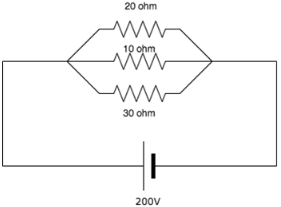Solution:

Explanation: I=V/R. Since in parallel circuit, voltage is same across all resistors. Hence across the 20 ohm resistor, V=200V so I=200/20=10A.

QUESTION: 13

In a parallel circuit, with a number of resistors, the voltage across each resistor is ________

Solution:

In parallel circuits, the current across the circuits vary whereas the voltage remains the same.

QUESTION: 14

Which is the most cost efficient connection?

Solution:

The advantage of series-connections is that they share the supply voltage, hence cheap low voltage appliances may be used.

QUESTION: 15

Calculate the total current in the circuit.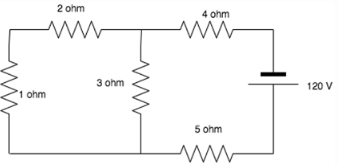Solution:

The 1 ohm and 2-ohm resistor are in series which is in parallel to the 3-ohm resistor.
The equivalent of these resistances is in series with the 4 ohms and 5-ohm resistor.
Total R= 10.5 ohm. I=V/R= 120/10.5= 11.42A.

QUESTION: 16

The voltage across the open circuit is?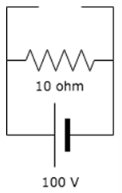Solution:

The voltage across all branches in a parallel circuit is the same as that of the source voltage. Hence the voltage across the 10 ohm resistor and the open circuit is the same=100V.

QUESTION: 17

The voltage across the short is?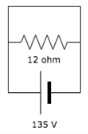Solution:

The voltage across a short is always equal to zero whether it is connected in seroes or parallel.

QUESTION: 18

If the current through the voltage in the circuit is 5A, find the value of x.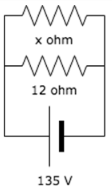Solution:

R=V/I. In this circuit I=5A and V=135V. Therefore, R=135/5=27 ohm.

QUESTION: 19

The currents in the three branches of a parallel circuit are 3A, 4A and 5A. What is the current leaving it?

Solution:

The total current leaving a node is the same as the current that enters it. Total I=I1+I2+I3=3+4+5=12A.

QUESTION: 20

The total resistance between A and B are?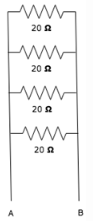Solution:

The resistors are connected in parallel, hence the equivalent resistance= 1/(1/20=1/20+1/20+1/20)=5ohm.

QUESTION: 21

Is it preferable to connect bulbs in series or in parallel?

Solution:

Bulbs are connected in parallel so that even if one of the bulbs blow out, the others continue to get a current supply.

QUESTION: 22

Calculate the total resistance between the points A and B.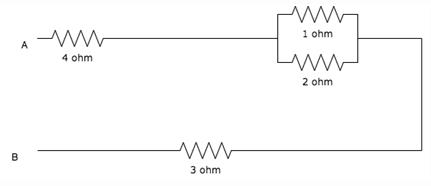Solution:

1 ohm in parallel with 2 ohm give 2/3 ohm equivalent which is in series with 4 ohm and 3 ohm so total resistance between A and B = 4 + 2/3 + 3 = 23/3 = 7.66 ohm.

QUESTION: 23

Calculate the equivalent resistance between A and B.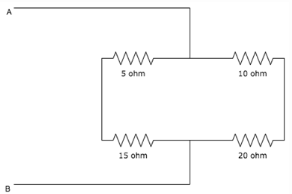Solution:

The 5 ohm and 15 ohm resistances are connected in series and 10 ohm and 20 ohm resistors are also connected in series. Therefore,20 ohm and 30 ohm are parallel with each other.

QUESTION: 24

Calculate the equivalent resistance between A and B.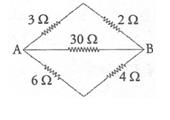Solution: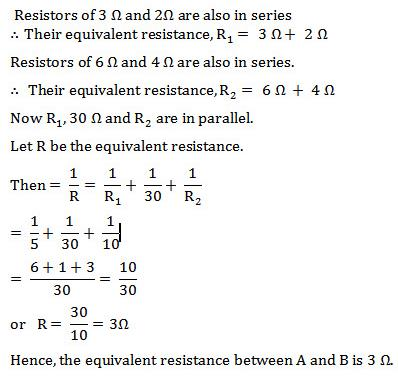QUESTION: 25

Batteries are generally connected in _____

Solution:

Batteries are generally connected in series so that we can obtain the desired voltage since voltages add up once they are connected in series.

QUESTION: 26

In a _________ circuit, the total resistance is greater than the largest resistance in the circuit.

Solution:

In series circuits the total resistance is the sum of all the resistance in the circuit, hence the total is greater than the largest resistance.

QUESTION: 27

In a ____________ circuit, the total resistance is smaller than the smallest resistance in the circuit.

Solution:

in a parallel circuit, the equivalent resistance=1/sum of the reciprocals of all the re-sistances in the circuit. Hence it is smaller than the smallest resistance in the circuit.

QUESTION: 28

What is the current through R1 and R2 in the following diagram?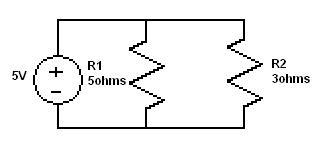Solution:

All are In parallel so voltage is same
IR1= 5/5 =1A
IR2 = 5/3 = 1.667A

QUESTION: 29

Calculate the equivalent resistance between A and B.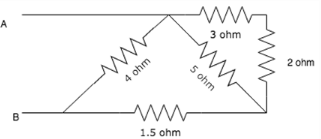Solution:

R=((2+3)||5)+1.5)||4.The 2 and the 3 ohm resistor are in series. The equiva-lent of these two resistors is in parallel with the 5 ohm resistor. The equivalent of these three resistances is in series with the 1.5 ohm resistor. Finally, the equivalent of these re-sistances is in parallel with the 4 ohm resistor.

QUESTION: 30

Calculate the equivalent resistance between A and B.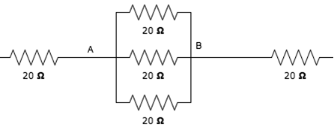Solution:

Between Point A and B;

R=20||20||20=6.67 ohm. The three 20 ohm resistors are in parallel and resistance is measured across this terminal. Net resistance is 6.67ohm.